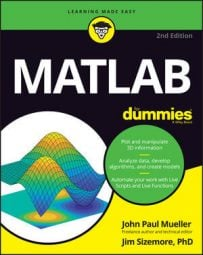##### MATLAB For DummiesOnce you know how to enter vectors and matrices in MATLAB, it’s time to see how to perform math using them. Adding and subtracting is a good place to start.

The essential rule when adding and subtracting vectors and matrices is that they must be the same size. You can’t add or subtract vectors or matrices of different sizes because MATLAB will display an error message. Use the following steps to see how to perform this task:

1. Type a=[1,2;3,4] and press Enter.

You see

```a =
1  2
3  4```
2. Type b=[5,6;7,8] and press Enter.

You see

```b =
5  6
7  8```
3. Type c = a + b and press Enter.

This step adds matrix a to matrix b. You see

```c =
6  8
10 12```
4. Type d = b - a and press Enter.

This step subtracts matrix b from matrix a. You see

```d =
4  4
4  4```
5. Type e=[1,2,3;4,5,6] and press Enter.

You see

```e =
1  2  3
4  5  6```

If you attempt to add or subtract matrix e from either matrix a or matrix b, you see an error message. However, the following step tries to perform the task anyway.

6. Type f = e + a and press Enter.

As expected, you see the following error message:

```Error using +
Matrix dimensions must agree.```

The error messages differ a little between addition and subtraction, but the idea is the same. The matrices must be the same size in order to add or subtract them.